Open in App
Not now

# Exporting Data from scripts in R Programming

• Last Updated : 01 Jun, 2020

So far the operations using R program are done on a prompt/terminal which is not stored anywhere. But in the software industry, most of the programs are written to store the information fetched from the program. One such way is to store the fetched information in a file. So the two most common operations that can be performed on a file are:

• Importing Data to R scripts
• Exporting Data from R scripts

#### Exporting Data from R Scripts

When a program is terminated, the entire data is lost. Storing in a file will preserve one’s data even if the program terminates. If one has to enter a large number of data, it will take a lot of time to enter them all. However, if one has a file containing all the data, he/she can easily access the contents of the file using a few commands in R. One can easily move his data from one computer to another without any changes. So those files can be stored in various formats. It may be stored in .txt(tab-separated value) file, or in a tabular format i.e .csv(comma-separated value) file or it may be on internet or cloud. R provides very easier methods to export data to those files.

#### Exporting data to a text file

One of the important formats to store a file is in a text file. R provides various methods that one can export data to a text file.

• `write.table()`: The R base function `write.table()` can be used to export a data frame or a matrix to a text file.

Syntax:
write.table(x, file, append = FALSE, sep = ” “, dec = “.”, row.names = TRUE, col.names = TRUE)

Parameters:
x: a matrix or a data frame to be written.
file: a character specifying the name of the result file.
sep: the field separator string, e.g., sep = “\t” (for tab-separated value).
dec: the string to be used as decimal separator. Default is “.”
row.names: either a logical value indicating whether the row names of x are to be written along with x, or a character vector of row names to be written.
col.names: either a logical value indicating whether the column names of x are to be written along with x, or a character vector of column names to be written.

Example:

 `# R program to illustrate ` `# Exporting data from R ` ` `  `# Creating a dataframe  ` `df ``=` `data.frame(  ` `  ``"Name"` `=` `c(``"Amiya"``, ``"Raj"``, ``"Asish"``),  ` `  ``"Language"` `=` `c(``"R"``, ``"Python"``, ``"Java"``),  ` `  ``"Age"` `=` `c(``22``, ``25``, ``45``)  ` `)  ` ` `  `# Export a data frame to a text file using write.table() ` `write.table(df, ` `            ``file` `=` `"myDataFrame.txt"``, ` `            ``sep ``=` `"\t"``, ` `            ``row.names ``=` `TRUE, ` `            ``col.names ``=` `NA) `

Output: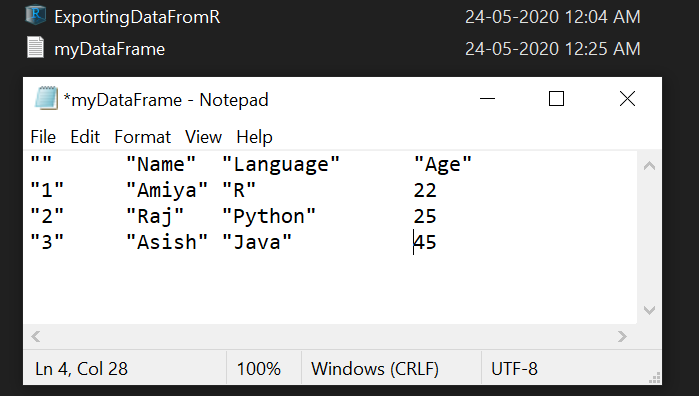• `write_tsv()`: This method is also used for to export data to a tab separated (“\t”) values by using the help of `readr` package.

Syntax: write_tsv(file, path)

Parameters:

file: a data frame to be written
path: the path to the result file

Example:

 `# R program to illustrate ` `# Exporting data from R ` ` `  `# Importing readr library ` `library(readr) ` ` `  `# Creating a dataframe  ` `df ``=` `data.frame(  ` `  ``"Name"` `=` `c(``"Amiya"``, ``"Raj"``, ``"Asish"``),  ` `  ``"Language"` `=` `c(``"R"``, ``"Python"``, ``"Java"``),  ` `  ``"Age"` `=` `c(``22``, ``25``, ``45``)  ` `)  ` ` `  `# Export a data frame using write_tsv()  ` `write_tsv(df, path ``=` `"MyDataFrame.txt"``) `

Output: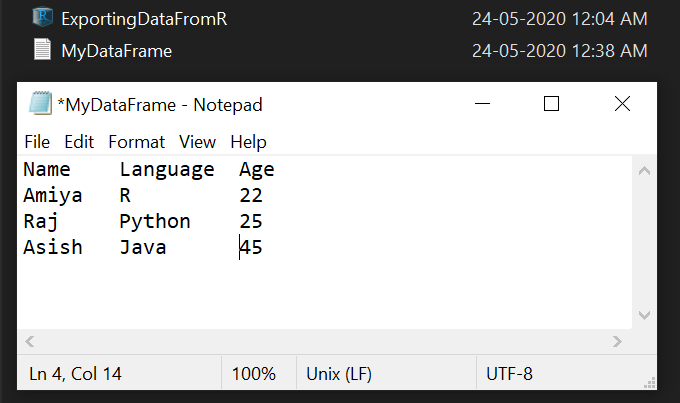#### Exporting data to a csv file

Another popular format to store a file is in a csv(comma-separated value) format. R provides various methods that one can export data to a csv file.

• `write.table()`: The R base function `write.table()` can also be used to export a data frame or a matrix to a csv file.

Syntax:
write.table(x, file, append = FALSE, sep = ” “, dec = “.”, row.names = TRUE, col.names = TRUE)

Parameters:
x: a matrix or a data frame to be written.
file: a character specifying the name of the result file.
sep: the field separator string, e.g., sep = “\t” (for tab-separated value).
dec: the string to be used as decimal separator. Default is “.”
row.names: either a logical value indicating whether the row names of x are to be written along with x, or a character vector of row names to be written.
col.names: either a logical value indicating whether the column names of x are to be written along with x, or a character vector of column names to be written.

Example:

 `# R program to illustrate ` `# Exporting data from R ` ` `  `# Creating a dataframe  ` `df ``=` `data.frame(  ` `  ``"Name"` `=` `c(``"Amiya"``, ``"Raj"``, ``"Asish"``),  ` `  ``"Language"` `=` `c(``"R"``, ``"Python"``, ``"Java"``),  ` `  ``"Age"` `=` `c(``22``, ``25``, ``45``)  ` `)  ` ` `  `# Export a data frame to a text file using write.table() ` `write.table(df, ` `            ``file` `=` `"myDataFrame.csv"``, ` `            ``sep ``=` `"\t"``, ` `            ``row.names ``=` `FALSE, ` `            ``) `

Output: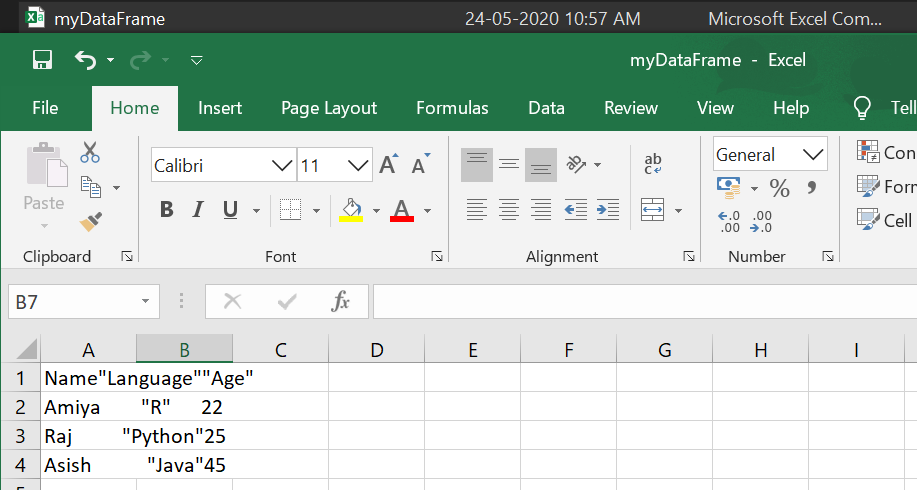• `write.csv()`: This method is recommendable for exporting data to a csv file. It uses “.” for the decimal point and a comma (“, ”) for the separator.

Example:

 `# R program to illustrate ` `# Exporting data from R ` ` `  `# Creating a dataframe  ` `df ``=` `data.frame(  ` `  ``"Name"` `=` `c(``"Amiya"``, ``"Raj"``, ``"Asish"``),  ` `  ``"Language"` `=` `c(``"R"``, ``"Python"``, ``"Java"``),  ` `  ``"Age"` `=` `c(``22``, ``25``, ``45``)  ` `)  ` ` `  `# Export a data frame to a text file using write.csv() ` `write.csv(df, ``file` `=` `"my_data.csv"``) `

Output: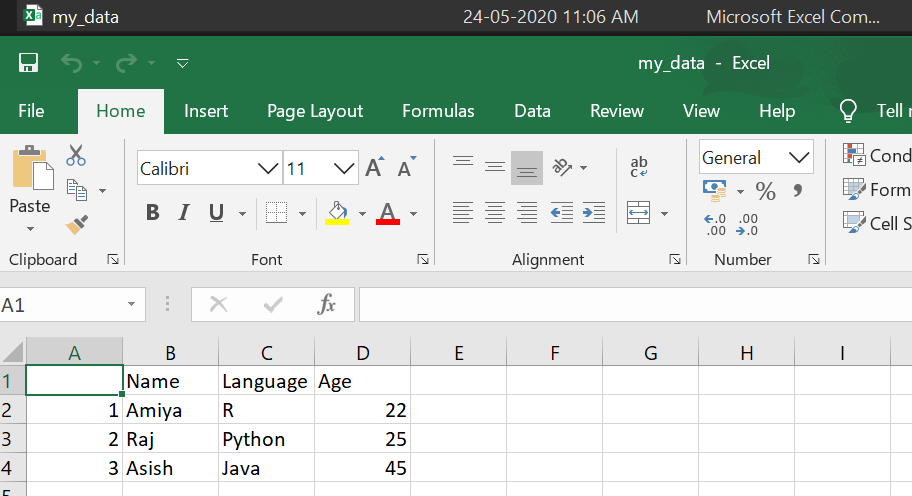• `write.csv2()`: This method is much similar as `write.csv()` but it uses a comma (“, ”) for the decimal point and a semicolon (“;”) for the separator.

Example:

 `# R program to illustrate ` `# Exporting data from R ` ` `  `# Creating a dataframe  ` `df ``=` `data.frame(  ` `  ``"Name"` `=` `c(``"Amiya"``, ``"Raj"``, ``"Asish"``),  ` `  ``"Language"` `=` `c(``"R"``, ``"Python"``, ``"Java"``),  ` `  ``"Age"` `=` `c(``22``, ``25``, ``45``)  ` `)  ` ` `  `# Export a data frame to a text file using write.csv2() ` `write.csv2(df, ``file` `=` `"my_data.csv"``) `

Output: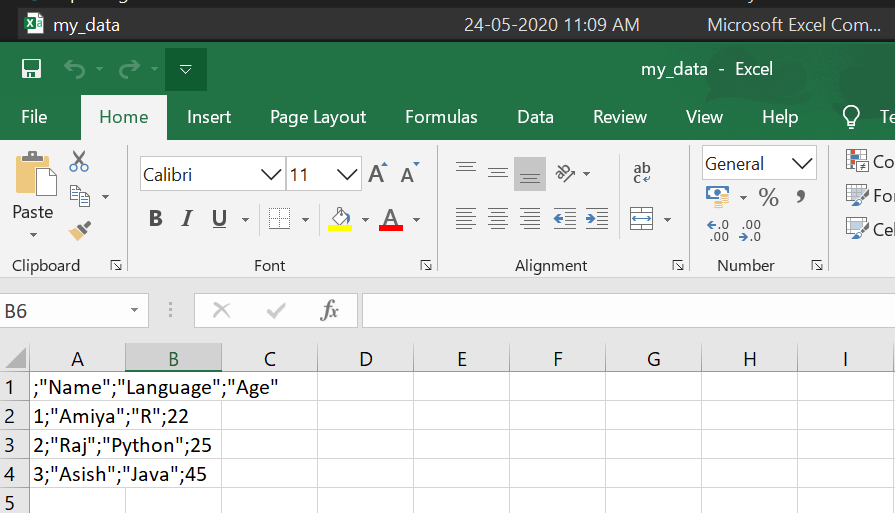• `write_csv()`: This method is also used for to export data to a comma separated (“, ”) values by using the help of readr package.

Syntax: write_csv(file, path)

Parameters:
file: a data frame to be written
path: the path to the result file

Example:

 `# R program to illustrate ` `# Exporting data from R ` ` `  `# Importing readr library ` `library(readr) ` ` `  `# Creating a dataframe  ` `df ``=` `data.frame(  ` `  ``"Name"` `=` `c(``"Amiya"``, ``"Raj"``, ``"Asish"``),  ` `  ``"Language"` `=` `c(``"R"``, ``"Python"``, ``"Java"``),  ` `  ``"Age"` `=` `c(``22``, ``25``, ``45``)  ` `)  ` ` `  `# Export a data frame using write_csv()  ` `write_csv(df, path ``=` `"MyDataFrame.csv"``) `

Output: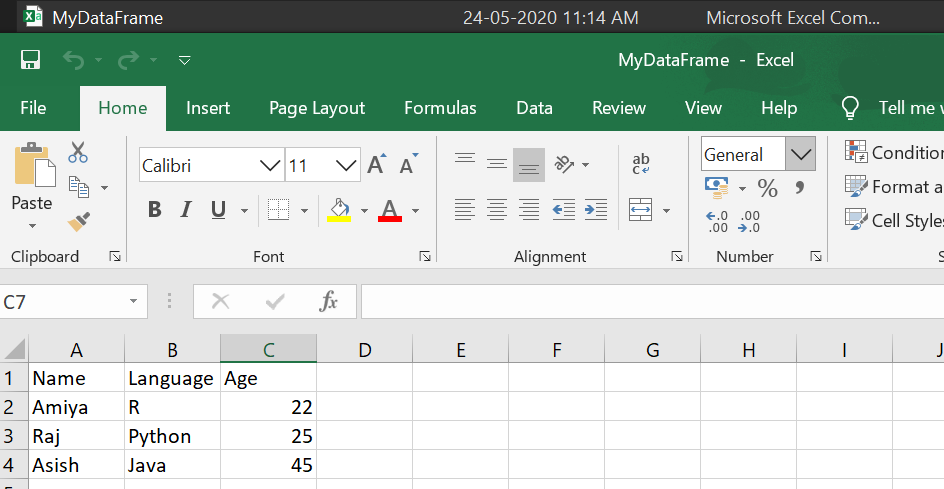My Personal Notes arrow_drop_up
Related Articles